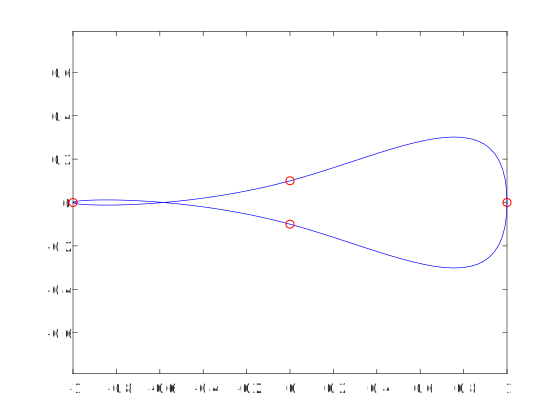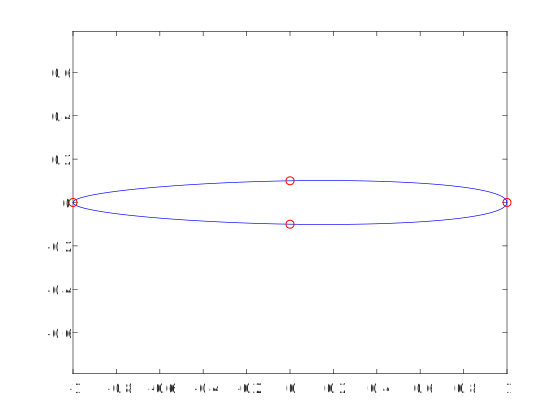# How to define endslopes for spline interpolation for 2-dimensional curves?

5 ビュー (過去 30 日間)
David 2019 年 3 月 4 日
While working with splines and trying to define some endslopes, I had some problems:
The example from matlab help worked fine:
x = pi*[0:.5:2];
y = [0 1 0 -1 0 1 0;
1 0 1 0 -1 0 1];
pp = spline(x,y);
yy = ppval(pp, linspace(0,2*pi,101));
plot(yy(1,:),yy(2,:),'-b',y(1,2:5),y(2,2:5),'or')
axis equal
But after that I wanted to try something like an ellipse:
x = pi*[0:.5:2];
y = [0 1 0 -1 0 1 0;
1 0 .1 0 -.1 0 1];
pp = spline(x,y);
yy = ppval(pp, linspace(0,2*pi,101));
plot(yy(1,:),yy(2,:),'-b',y(1,2:5),y(2,2:5),'or')
axis equalI figured out that I had to change the endslopes to 0.15 to obtain the expected ellipse:
x = pi*[0:.5:2];
y = [0 1 0 -1 0 1 0;
.15 0 .1 0 -.1 0 .15];
pp = spline(x,y);
yy = ppval(pp, linspace(0,2*pi,101));
plot(yy(1,:),yy(2,:),'-b',y(1,2:5),y(2,2:5),'or')
axis equalNow I was wondering, whether there is any simple way (formula) with which you can choose the endslopes rather than just "trying"

サインインしてコメントする。

### カテゴリ

Find more on Splines in Help Center and File Exchange

R2018b

### Community Treasure Hunt

Find the treasures in MATLAB Central and discover how the community can help you!

Start Hunting!